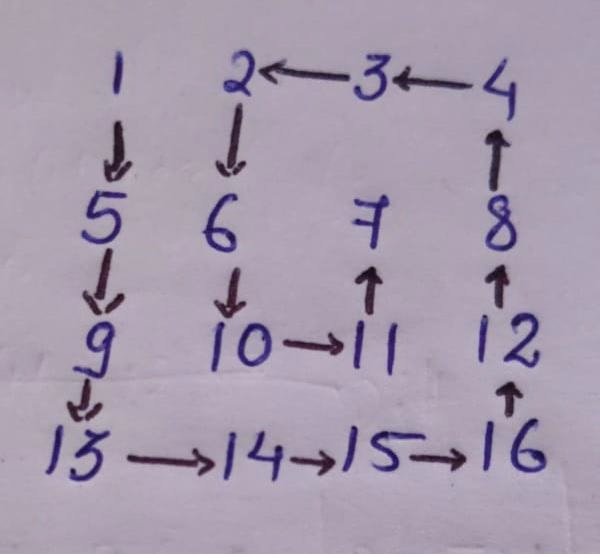# Print a given matrix in counter-clockwise spiral form in C++

C++Server Side ProgrammingProgramming

In this problem, we are given a 2-dimensional matrix. And our task is to print the elements of the matrix in a counter-clockwise spiral from.

Counterclockwise Spiral Form − It is a spiral traversal which starts from top-left and the goes in the counter-clockwise direction to first bottom-right-up-left.The counter-clockwise traversal will be 1 5 9 13 14 15 16 12 8 4 3 2 6 10 11 7.

Let’s take an example to understand the problem

Input:
2 4 6
1 7 9
5 0 3
Output: 2 1 5 0 3 9 7

To solve this problem, we will be using four loops and each in one direction and initializing their direction and traversing accordingly.

## Example

Program to show the implementation of our solution

Live Demo

#include <bits/stdc++.h>
using namespace std;
#define R 3
#define C 3
void printCounterClockwiseSpiral(int m, int n, int matrix[R][C]){
int i, k = 0, l = 0;
int count = 0;
int total = m * n;
while (k < m && l < n){
if (count == total)
break;
for (i = k; i < m; ++i){
cout<<matrix[i][l]<<" ";
count++;
}
l++;
if (count == total)
break;
for (i = l; i < n; ++i){
cout<<matrix[m - 1][i]<<" ";
count++;
}
m--;
if (count == total)
break;
if (k < m){
for (i = m - 1; i >= k; --i){
cout<<matrix[i][n - 1]<<" ";
count++;
}
n--;
}
if (count == total)
break;
if (l < n){
for (i = n - 1; i >= l; --i){
cout<<matrix[k][i]<<" ";
count++;
}
k++;
}
}
}
int main() {
int mat[R][C] = {
{ 1, 2, 3 },
{ 4, 5, 6 },
{ 7, 8, 9}
};
cout<<"Conter Clockwise Spiral from of the matrix is :\n";
printCounterClockwiseSpiral(R, C, mat);
return 0;
}

## Output

Counter Clockwise Spiral from of the matrix is −

1 4 7 8 9 6 3 2 5